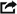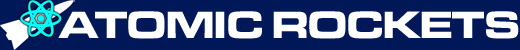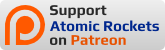## MissionsPlanetary Transfer Calculator: This is impressive! It calculates various types of transfers (including torchship brachistochrone transfers). It can calculate ballistic transfers between planets and moons, and powered (constant acceleration) transfers between stars (including effects of relativity). It can also calculate propagation delay due to the absolute speed of light between planets and moons.

Back-of-the-envelope Orbital Transfer Calculator is an on-line calculator for Hohmann trajectories (only) created by Pete Wildsmith. It is basically a wrapper around Erik Max Francis' BOTE Python library. There are some simplifications which reduce the accuracy a bit, read the docs at the "BOTE Python library" link under "Limitations" for details.

Orbital Energy: Calculate the orbital energy required to launch a payload from the surface into the desired orbit. By Ian Mallett.

EasyPorkchop: Calculate "pork-chop" or C3 plot for various missions, so you can chose a mission within the delta-V capacity of your spacecraft. It uses a concept called hyperbola's velocity at infinity or Vinf. For an easy explanation of Vinf, go here.

Basic Hohmann Mission Spreadsheet (format Microsoft Excel 97-2003 XLS): For a Hohmann trip from low orbit around Planet Start to low orbit around Planet Dest, calculate total delta-V, Transit time, time delay between launch windows, and time delay between arrival and launch window opening for return trip. Implements menthod outlined here. This spreadsheet was written by me and possibly contains mistakes. Use at your own risk.

Basic Hohmann Mission Spreadsheet (format Libre Office Calc ODS): Same spreadsheet as above, but in Libre Office format.

## Spacecraft Design

### Structure

TransHab Module Calculator: Calculates the dimensions of an inflatable TransHab crew module. By Rob Davidoff.

Structural Mass Calculator: Estimates the structural mass of a spacecraft, using the method found here. By Eric Rozier.

Multi-Stage Rocket Delta-V Optimizer: Calculate the delta V increase created by staging your spacecraft. By Ian Mallett.

### Rocket Engine

Performance of Expansion-Type Rocket Engines: given propellant density, propellant heat capacity ratio, engine chamber pressure, engine chamber temperature, and exit pressure, this will calculate the engine's exhaust velocity and specific impulse. By Ian Mallett

Thruster Calculator: Simulates spacecraft thrust, given rocket engine type and mission profile. By Eric Rozier.

Delta-V Calculator: Given the rocket's wet mass, dry mass, and specific impulse/exhaust velocity calculate the rocket's delta-V. By Joseph J. Strout.

The Rocket Equation: Calculates the delta-V equation. Given any three of the values it calculates the value of the fourth. Original calculator by Trent Waddington, but the website vanished. The link now points to a mirror site created by Alastair Cranston

Panel or Droplet Radiator Calculator by Ian Mallett. He says for droplet radiators this calculator does not simulate occlusion between droplets.

Droplet Radiator Calculator: Given a droplet radiator design, calculate required area to dissapate desired waste heat level. Discussion here. By Eric Rozier. Note: Ian Mallett says that the surface area is erroneously reported as being in MW (it's actually in kW) and that the temperature of space is not accounted for.

Coolant Calculator: Given a radiator's coolant type and amount, simulate the radiator system. By Eric Rozier.

### Misc

Mission Calculator: Given crew size and mission length, calculate the amount of required consumables. By Eric Rozier.

Ship Decompression: Calculate how fast the air will rush out a meteor hole in the hull. By Ian Mallett.

Relativistic Star Ship Calculator: Calculates times to reach distant points by a relativisitic star ship, accelerating continuously. By Stephen R. Schmitt.

Artificial Gravity: Calculate the parameters of a centrifugal artificial gravity system. By Ian Mallett.

SpinCalc: Calculate the parameters of a centrifugal artificial gravity system. By Theodore W. Hall.

Radiation Exposure Calculator: Simulates exposing a simulated human to a radiation dosage of your choice. By Ian Mallett.

Black Hole Parameters: Calculate the properties of a black hole. By Ian Mallett.

Hawking Radiation Calculator: Calculate the radiation emitted by a Hawking black hole. By Jim Wisniewski.

## Weapons

### Nuclear Weapons

Nuclear Weapon Calculator: Models the effect of various weapon sizes on user selected armor samples. By Eric Rozier.

Nuclear Weapon Effects Calculator: For various nuclear weapon yields, calculates the thermal radius, air blast radius, ionizing radiation radius, and other values. By Michael Wong

Nuclear Bomb Effects Computer: For various nuclear weapon yields, calculates blast and fallout values, and displays them on a pseudo-slide rule. By John Walker

### Laser Weapons

Laser Weapon Calculator: Models the effect of various types of lasers on user selected armor samples. By Eric Rozier.

Pulse Laser Calculator: Estimates the effects of pulsed laser blasts on a target. By Luke Campbell.

Beam Laser Calculator: Estimates the effects of a beam laser on a target. By Luke Campbell.

### Kinetic Weapons

Kinetic Weapon Calculator: Models the effect of various types of projectile weapons on user selected armor samples. By Eric Rozier.

Relativistic Kinetic Energy: Kinetic energy of projectiles moving close to the speed of light.

### Point Defense

Point Defense Hit Probabilities - Kinetic CIWS: Estimates hit probabilities of kinetic point defence on various hostile missile sizes. By Eric Rozier.

Point Defense Hit Probabilities - Missile CIWS: Estimates hit probabilities of missile based point defence on various hostile missile sizes. By Eric Rozier.

## Asteroid Strike

Earth Impact Effects Program: Estimates the effects of an asteroid striking Earth. By Robert Marcus, H. Jay Melosh, and Gareth Collins.

Impact Calculator: Estimates the effects of an asteroid striking Earth. Includes Google Maps so you can plot the size of the crater. By Edward Gomez and Jon Yardley.

Impact Calculator: Estimates the effects of identical asteroids striking Earth and Luna.

Solar System Collisions: Estimates the effects of an asteroids striking the planet of your choice. By Dr. Douglas P. Hamilton

## Worldbuilding

SF CalcSheet: free open-source science fiction calculation spreadsheet for the free open-source LibreOffice. Calculates:

• Constants: Collection of physical constants used in the spreadsheet's internals.
• Conversion: Unit conversion
• Light years <-> parsec
• km <-> AU
• m/s <-> km/h
• Temperature
• Length (SI <-> USCS)
• Area (SI <-> USCS)
• Volume (SI <-> USCS)
• Mass/weight (SI <-> USCS)
• Star: Star related calculators.
• Magnitude <-> luminosity
• Magnitude/distance
• Luminosity difference
• Star color
• Star properties
• Planet: Planet calculators.
• Mass converter
• Physical properties
• Orbital properties
• Tidal forces
• Planetary habitability
• Population density
• City area
• Satellite: Calculators pertaining to natural satellites, i.e. moons.
• Satellite appearance
• Satellite orbit
• Compact: Compact object calculators.
• Schwarzschild black hole properties
• Impact: Impact calculators.
• Impact and crater
• Neutron matter explosion
• Life: Calculators related to living beings.
• Creature body weight
• Vegetation color
• Structure: Calculators for large structures.
• Structure properties and resources
• Vehicle: Vehicle calculators.
• Vehicle mass and personnel
• Travel: Travel calculators.
• FTL factor
• FTL travel time
• Time dilation
• Uniform acceleration
• Terrestrial travel time

## Atomic Rockets notices

This week's featured addition is SPIN POLARIZATION FOR FUSION PROPULSION

This week's featured addition is INsTAR

This week's featured addition is NTR ALTERNATIVES TO LIQUID HYDROGEN

### Support Atomic Rockets